Courses

Revision Notes - Electronic Devices Notes | Study Mock Test Series for JEE Main & Advanced 2022 - JEE

JEE: Revision Notes - Electronic Devices Notes | Study Mock Test Series for JEE Main & Advanced 2022 - JEE

The document Revision Notes - Electronic Devices Notes | Study Mock Test Series for JEE Main & Advanced 2022 - JEE is a part of the JEE Course Mock Test Series for JEE Main & Advanced 2022.
All you need of JEE at this link: JEE

THERMIONIC EMISSION
Thermionic emission is the phenomenon in which electrons are emitted by a metal contains free electrons which behave like the molecules of a perfect gas.

RICHARDSON EQUATION
I = AT1/2e-b/T
Here I is the thermionic current density in amp per sq meter. T is the temperature on kelvin scale, A and b being constants.
A = ne√k/2πm, b = e?/k
Here, n is the number of electrons per unit volume, e is the charge on electron, m is the mass of electron, k is the Boltzmann’s constant and ? is the potential barrier of the metal.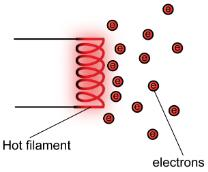There are three types of energy bands in a solid viz.
(a)Valence energy band
(b)Conduction energy band
(c)Forbidden energy gap.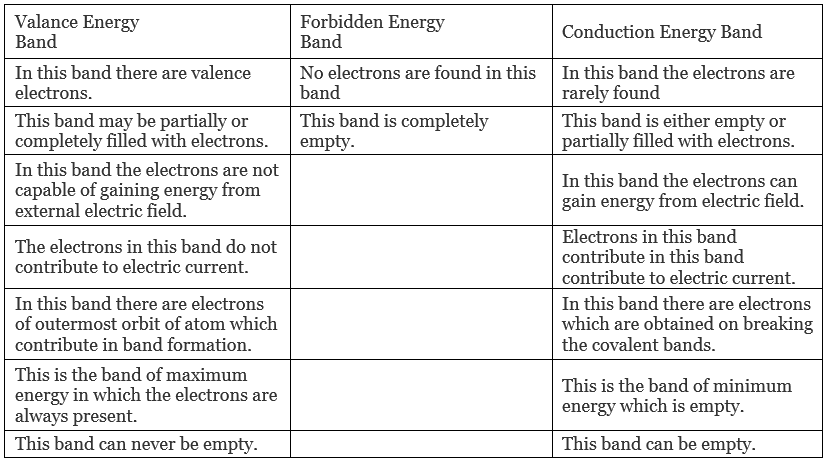Energy gap or Band gap (Eg)
(a) The minimum energy which is necessary for shifting electrons from valence band to conduction band is defined as band gap (Eg)
(b) The forbidden energy gap between the valence band and the conduction band is known as band gap (Eg). i.e. Eg = E– Ev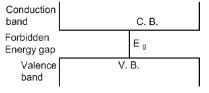(c) As there are energy levels f electrons in an atom, similarly there are three specific energy bands for the electrons in the crystal formed by these atoms as shown in the figure,
(d)Completely filled energy bands: The energy band, in which maximum possible numbers of electrons are present according to capacity is known as completely filled bank.
(e) Partially filled energy bands: The energy band, in which number of electrons present is less than the capacity of the band, is known as partially filled energy band.
(f) Electric conduction is possible only in those solids which have empty energy band or partially filled energy band.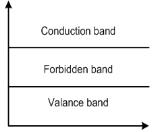Various types of solids
On the basis of band structure of crystals, solids are divided in three categories.
(a) Insulators
(b) Semi-conductors
(c) Conductors.

The number of electrons or cotters is given by
ni = pi = AT3/2 e - Eg/2KT
i.e. on increasing temperature, the number of current carriers increases.

The semiconductors are of two types.
(a) Intrinsic or pure semiconductors
(b) Extrinsic or dopes semiconductors

Difference between Intrinsic and Extrinsic semiconductors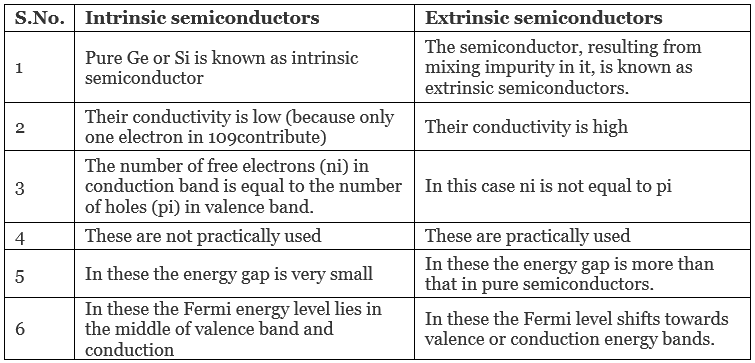Child’s law: I= KVp3/2, K is the proportionality constant.

Work function: Work function of a metal is the amount of energy required to pull an electron from the surface of metal to a distant position.

Diode: It is a vacuum tube containing two electrodes, an emitter and a collector.

Diode as a rectifier: Rectification is the process of converting the alternating current into a unidirectional current.
Rectification can be done by making use of a diode. A diode affecting rectification is said to be acting as a rectifier.

DIODE RESISTANCE
(a) Static plate resistance
(i) Rp = Vp/Ip
(ii) Rp ∝ (Vp)-1/2
(iii) Rp ∝ (Ip)-1/3
(b) Dynamic plate resistance:-
(i) rp = (ΔVp/ΔIp)
(ii) rp∝Vp-1/2
(iii) rp∝Ip-1/2

Half-wave rectifier: It is the type of rectification in which only one half of the input a.c. is translated into the output.

Full-wave rectifier: A rectifier in which current flows through the load for both the halves of input a.c. is called half-wave rectifier.

TRIODE
It is a vacuum tube containing three elements namely plate, filament and grid.

Triode constants
(a) Plate resistance (rp): It is defined as the ratio between changes in plate potential keeping grid potential constant to the corresponding change in plate current.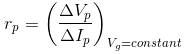(b) Mutual Inductance (gm): (Trans-conductance):- It is defined as the ratio between change in plate current  to the change in grid potential keeping plate potential constant required to bring about that change in current.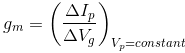(c) Amplification factor (μ): it is defined as the ratio between change in plate potential keeping grid potential constant to the change in grid potential keeping plate potential constant, in order to bring about same change in plate current.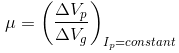(d) Relation between μ, rp and gm: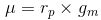(e) rp ∝Ip-1/3
(f) gm∝Ip1/3

Triode as an amplifier: Process of increasing the amplitude of the input signal is called amplification. A triode affecting an increase in the amplitude of a signal is said to be acting as an amplifier.

Voltage gain
It is the ratio between the output voltage (voltage across the load resistance RL) to the signal voltage.
Voltage gain = V0/e= μRL/RL+rp = μ/[1+(rp/RL)]
This indicates that the voltage gain depend upon the load resistance. For RL=∞, voltage gain is equal to the amplification factor.
(a) Ip = (μVg/RL+rp)
(b) A =μRL/RL+rp
(c) Amax = μ
(d) μ = A [1+(rp/RL)]
(e) A = μ/2   if RL = rp

Cut off voltage
Vg = -(Vp/μ)

Plate current equation
Ip = K[Vg+(Vp/μ)]3/2

Triode as an oscillator:- A triode producing high frequency oscillating waves, of constant amplitude, is said to be acting as an oscillator.
Frequency of oscillation waves (f):- f = 1/2π√LC
Here L is the inductance and C is the capacitance.

Majority charge carrier
(a) For N-type semiconductor:- electron
(b) For P-type semiconductor:- hole

Electrical conductivity of semiconductors:-
Intrinsic semiconductors:-
(a) σ = e(neμe + nhμh)
Here, ne is the electron density, nh is the hole density,  is the electric mobilities μe and μh is the hole mobilities.
(b)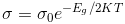Extrinsic semiconductor
(a) n-type:- σ = eneμe
(b) p-type:- σ = ennμn

Transistor
(a) IE = IC+IB       (IB << IE, IB<<IC)
(b) Current gains:-
α = IC/IE, αac = ΔIC/ΔIE
β = IC/IB, βac = ΔIC/ΔIB
(c) Relation between α and β:-
α = β/[1+ β]
or
β = α/[1-α]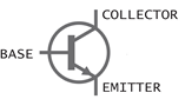The document Revision Notes - Electronic Devices Notes | Study Mock Test Series for JEE Main & Advanced 2022 - JEE is a part of the JEE Course Mock Test Series for JEE Main & Advanced 2022.
All you need of JEE at this link: JEEUse Code STAYHOME200 and get INR 200 additional OFF Use Coupon Code

Top Courses for JEEMock Test Series for JEE Main & Advanced 2022

2 videos|258 docs|160 tests

Top Courses for JEETrack your progress, build streaks, highlight & save important lessons and more!

,

,

,

,

,

,

,

,

,

,

,

,

,

,

,

,

,

,

,

,

,

;